# Common Core: High School - Geometry : Congruence

## Example Questions

### Example Question #4 : Rotations And Reflections Of Rectangles, Parallelograms, Trapezoids, And Regular Polygons: Ccss.Math.Content.Hsg Co.A.3

If the rectangle is rotated clockwise around the origindegrees, what is the resulting image?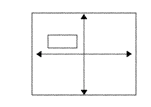None of the answers.Explanation:

To rotate the rectangular object around the origin, first recall the definition for a rotation and origin.

Rotation: To rotate an object either clockwise or counter clockwise around a center point. In this particular case the center point is the origin or the pointwhere the x and y axis intersect.

Looking at the original image and making one 90 degree rotation around the origin results in the following.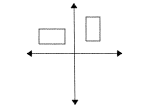When rotating, the bottom right point will become the bottom left point, the top right point becomes the bottom right point, the left bottom point becomes the top left point, and the left top point becomes the top right point.

Now, rotating it anotherdegrees to get to thedegree rotated image, results in the following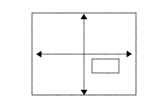### Example Question #5 : Rotations And Reflections Of Rectangles, Parallelograms, Trapezoids, And Regular Polygons: Ccss.Math.Content.Hsg Co.A.3

If the rectangle is rotated clockwise around the origindegrees, what is the resulting image?None of the answers.Explanation:

To rotate the rectangular object around the origin, first recall the definition for a rotation and origin.

Rotation: To rotate an object either clockwise or counter clockwise around a center point. In this particular case the center point is the origin or the pointwhere the x and y axis intersect.

Looking at the original image and making one rotation around the origin results in the following.When rotating, the bottom right point will become the bottom left point, the top right point becomes the bottom right point, the left bottom point becomes the top left point, and the left top point becomes the top right point. The visual representation for this rotation is as follows.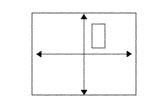### Example Question #6 : Rotations And Reflections Of Rectangles, Parallelograms, Trapezoids, And Regular Polygons: Ccss.Math.Content.Hsg Co.A.3

If the rectangle is rotated clockwise around the origindegrees, what is the resulting image?Explanation:

To rotate the rectangular object around the origin, first recall the definition for a rotation and origin.

Rotation: To rotate an object either clockwise or counter clockwise around a center point. In this particular case the center point is the origin or the pointwhere the x and y axis intersect.

Looking at the original image and making onedegree rotation around the origin results in the following.When rotating, the bottom right point will become the bottom left point, the top right point becomes the bottom right point, the left bottom point becomes the top left point, and the left top point becomes the top right point.

Now, rotating it anotherdegrees to get to thedegree rotated image, results in the followingFrom here one final rotation must occur to reachdegrees.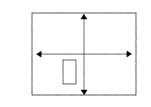### Example Question #7 : Rotations And Reflections Of Rectangles, Parallelograms, Trapezoids, And Regular Polygons: Ccss.Math.Content.Hsg Co.A.3

If the rectangle is rotated clockwise around the origindegrees, what is the resulting image?Explanation:

To rotate the rectangular object around the origin, first recall the definition for a rotation and origin.

Rotation: To rotate an object either clockwise or counter clockwise around a center point. In this particular case the center point is the origin or the pointwhere the x and y axis intersect.

Looking at the original image and making one 90 degree rotation around the origin results in the following.When rotating, the bottom right point will become the bottom left point, the top right point becomes the bottom right point, the left bottom point becomes the top left point, and the left top point becomes the top right point.

Now, rotating it anotherdegrees to get to thedegree rotated image, results in the followingFrom here another rotation must occur to reachdegrees.Lastly, complete one moredegree rotation to land at thedegree mark. Notice that rotating 360 degrees lands the image back at its original spot.### Example Question #31 : High School: Geometry

Determine whether the statement is true or false:

An equilateral diamond when rotateddegrees around its center results in the reflected image of two isosceles triangle.

True

False

True

Explanation:

To determine whether this statement is true or false first recall what arotation and what a reflection is.

Reflection: To flip the orientation of an object over a specific line or function.

Rotation: To rotate an object either clockwise or counter clockwise around a center point.

Now looking at the statement, draw images that depict the 90 degree rotation and the reflection of the original image to verify the truth of the statement.

"An equilateral diamond when rotated 90 degrees around its center results in the reflected image of two isosceles triangle."

The original equilateral diamond is: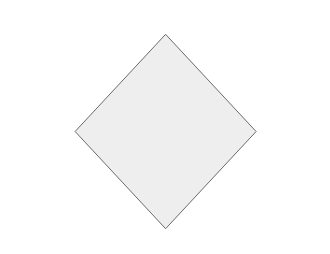The diamond rotateddegrees: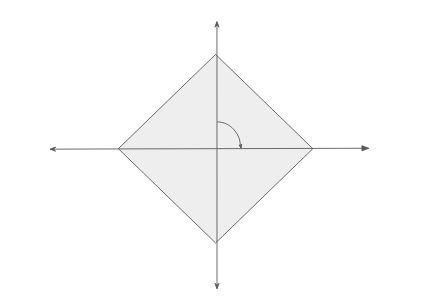The reflection of an isosceles triangle.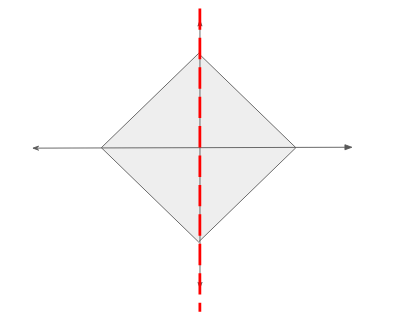Therefore, the statement is true.

### Example Question #32 : High School: Geometry

Determine whether the statement is true or false:

An equilateral diamond when rotateddegrees around its right most corner results in the reflected image of the original diamond.

False

True

True

Explanation:

To determine whether this statement is true or false first recall what arotation and what a reflection is.

Reflection: To flip the orientation of an object over a specific line or function.

Rotation: To rotate an object either clockwise or counter clockwise around a center point.

Now looking at the statement, draw images that depict thedegree rotation and the reflection of the original image to verify the truth of the statement.

"An equilateral diamond when rotateddegrees around its right most corner results in the reflected image of the original diamond."

The original equilateral diamond with rotation ofdegrees on its right most corner is as follows: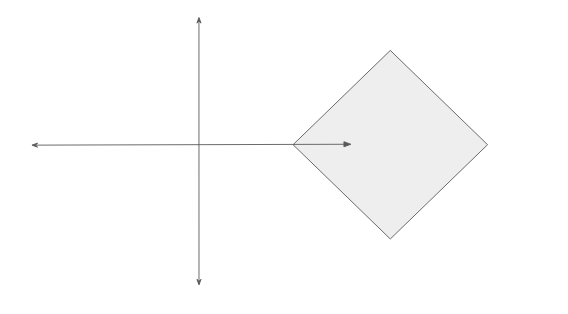The rotated image results in the reflected original image.

Therefore, the statement is true.

### Example Question #1 : Rotation, Reflection, And Transformation Definitions: Ccss.Math.Content.Hsg Co.A.4

Two pencils are placed equidistance away from one another on a table. If these two pencils are parallel, what is the result after they are reflected.

The two pencils are now perpendicular.

The two pencils are still parallel.

None of the answers.

The two pencils do not move.

The two pencils are located on the same line.

The two pencils are still parallel.

Explanation:

Before solving this problem recall the definition of a reflection.

Reflection: The transformation of each and every point on a line or figure across some line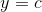whereis some constant or linear function.

Applying this definition to the situation at hand result in the following depiction. The pencils are represented by the blue and black lines and the reflection line is the red dashed line. After the reflection occurs, the pencils essentially swap positions. Since the pencils were parallel to start with, they remain parallel after the reflection occurred.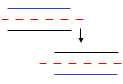Therefore, the correct answer is,

"The two pencils are still parallel."

### Example Question #2 : Rotation, Reflection, And Transformation Definitions: Ccss.Math.Content.Hsg Co.A.4

Given a circle that is divided intoequal pieces, what is the number of rotations that can occur to keep symmetry?

Twelve

Three

None of the answers.

Five

Six

Six

Explanation:

Drawing the described circle is the first step in answering this given question.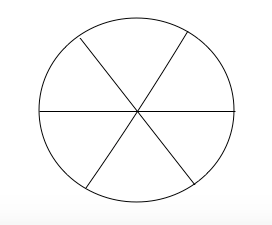To calculate the number of symmetrical rotations, take one piece and rotate it clockwise until it results in the exact image as originally started with. Since the circle is divided into six equal pieces that means that rotating one of the pieces can be done six different times and still keep rotational symmetry, which also means keeping the angles of each piece the same and returning to the original image.

Therefore the correct answer is six.

### Example Question #31 : Congruence

Given a regular polygon that hassides, calculate the order of rotational symmetry.Explanation:

For this particular question recall what rotational symmetry means. For an object to achieve rotational symmetry it must return to its original form. This can be accomplished by rotating the figure or equal parts of the figure in either the clockwise or non clockwise direction.

For this particular problem, it is stated that the polygon is "regular" meaning all sides and angles are equal to one another. It is also said that the polygon hassides. This means that the figure can be rotatedtimes to return to its original form.

Therefore, to obtain rotational symmetry of a regular polygon, it must have an order equal to the number of sides.

Thus, the answer is.

### Example Question #31 : Congruence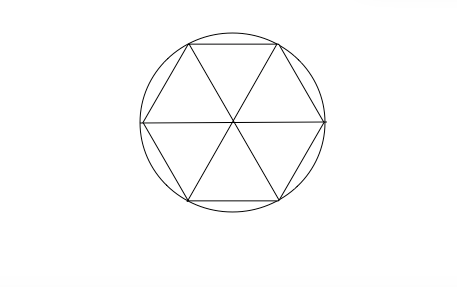Looking at the above image, calculate the order of rotational symmetry of the polygon that is inscribed in the circle.Explanation:

For this particular question recall what rotational symmetry means. For an object to achieve rotational symmetry it must return to its original form. This can be accomplished by rotating the figure or equal parts of the figure in either the clockwise or non clockwise direction.

For this particular problem, look at the following image.The polygon has six sides. This means that the figure can be rotatedtimes to return to its original form.

Therefore, to obtain rotational symmetry of a regular polygon, it must have an order equal to the number of sides.

Thus, the answer is.

### All Common Core: High School - Geometry Resources